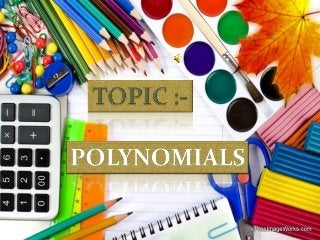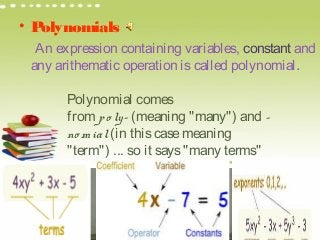Diese Präsentation wurde erfolgreich gemeldet.

# polynomial ppt for class 9×

6 von 19
6 von 19

# polynomial ppt for class 9

best of all from NITISH

best of all from NITISH

### polynomial ppt for class 9

1. 1. WE THE STUDENTS OF CLASS IX HAVE TRIED OUR BEST TO DO THE PRESENTATION IN SUCH A WAY THAT THIS REPRESENTATION WOULD BE THE BEST ONE
2. 2.  In mathematics, a polynomial is an expression consisting of variables (or indeterminate) and coefficients, that involves only the operations of addition, subtraction, multiplication, and non-negative integer exponents. An example of a polynomial of a single indeterminate (or variable), x, is x2 âˆ’ 4x + 7, which is a quadratic polynomial.  Polynomials appear in a wide variety of areas of mathematics and science. For example, they are used to form polynomial equations, which encode a wide range of problems, from elementary word problems to complicated problems in the sciences; they are used to define polynomial functions, which appear in settings ranging from basic chemistry and physics to economics and social science; they are used in calculus and numerical analysis to approximate other functions. In advanced mathematics, polynomials are used to construct polynomial rings and algebraic varieties, central concepts in algebra and algebraic geometry.
3. 3. WE HAVE TRIED OUR BEST TO PREPARE THE PROJECT. WE WANT TO THANKS ALL THE MEMBERS OF OUR GROUP FOR HAVING A GOOD RESPOND FOR THE PROJECT WHICH INSPIRED ALL.AT THE BUT NOT THE LEAST….WE RESPECTFULLY THANKS OUR TEACHER FOR GIVING SOME IDEA ABOUT THE PROJECT.
4. 4. • Polynomials  An expression containing variables, constant and any arithematic operation iscalled polynomial. Polynomial comes from po ly- (meaning "many") and - no mial (in thiscasemeaning "term") ... so it says"many terms"
5. 5. • Polynomialscontain threetypesof terms:- (1) monomial :- A polynomial with oneterm. (2) binomial :- A polynomial with two terms. (3) trinomial :- A polynomial with threeterms.
6. 6. • Degreeof polynomial :- thehighest power of the variablein apolynomial istermed asthedegreeof polynomial. • Constant polynomial :- A polynomial of degreezero is called constant polynomial. • Linear polynomial :- A polynomial of degreeone. • E.g. :-9x + 1 • Quadratic polynomial :- A polynomial of degree two. E.g. :-3/2y² -3y + 3 • Cubic polynomial :- A polynomial of degreethree. • E.g. :-12x³ -4x² + 5x +1 • Bi – quadratic polynomial :- A polynomial of degree four. • E.g. :- 10x – 7x ³+ 8x² -12x + 20
7. 7. • . Standard Form • The Standard Form for writing apolynomial isto put thetermswith thehighest degreefirst. • Example: Put this in Standard Form: 3x2  - 7 + 4x3  + x6 Thehighest degreeis6, so that goesfirst, then 3, 2 and then theconstant last: x6  + 4x3  + 3x2  - 7
8. 8. • Let p(x) beany polynomial of degree greater than or equal to oneand let a beany real number. If p(x) isdivided by linear polynomial x-athen the reminder isp(a). • Proof :- Let p(x) beany polynomial of degreegreater than or equal to 1. suppose that when p(x) isdivided by x-a, the quotient isq(x) and thereminder isr(x), i.g; p(x) = (x-a) q(x) +r(x) Remindertheorem
9. 9. Sincethedegreeof x-ais1 and thedegreeof r(x) is lessthan thedegreeof x-a,thedegreeof r(x) = 0. Thismeansthat r(x) isaconstant .say r. So , for every valueof x, r(x) = r. Therefore, p(x) = (x-a) q(x) + r In particular, if x = a, thisequation givesus p(a) =(a-a) q(a) + r Which provesthetheorem.
10. 10. Let p(x) beapolynomial of degreen > 1 and let abeany real number. If p(a) = 0 then (x-a) isafactor of p(x). PROOF:-By thereminder theorem , p(x) = (x-a) q(x) + p(a). FactorTheorem
11. 11. 1. If p(a) = 0,then p(x) = (x-a) q(x), which showsthat x-aisafactor of p(x). 2. Sincex-aisafactor of p(x), p(x) = (x-a) g(x) for samepolynomial g(x). In thiscase, p(a) = (a-a) g(a) =0
12. 12. Therefore our beauTiful projecT ends hope This projecT would help us To geT beTTer marks, which has became a greaT Task for us, in The pasT
13. 13.  We have collected the information from books and reference books to present the project successfully in the presentation. The clip art photos used in the Power Point presentation are brought from the world wide web.  THANK YOU
14. 14. THANKYOU